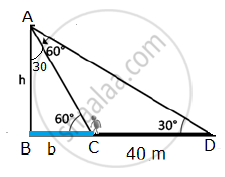SSC (Marathi Semi-English) 10thMaharashtra State Board
Share

# A person standing on the bank of river observes that the angle of elevation of the top of a tree standing on the opposite bank is 60° - SSC (Marathi Semi-English) 10th - Geometry

#### Question

A person standing on the bank of river observes that the angle of elevation of the top of a tree standing on the opposite bank is 60°. When he moves 40 m away from the bank, he finds the angle of elevation to be 30°. Find the height of the tree and width of the river. (sqrt 3=1.73)

#### Solution

Let AB = height of the tower = h metres
In the right angled ΔABC and right angled ΔABD,tan60^@=h/(BC)=sqrt(3) rArr BC=h/sqrt(3)

tan30^@=h/(BD)=1/sqrt(3) rArr BD=hsqrt(3)

Now, BD – BC = 40

hsqrt(3)-h/sqrt(3)=40

(3h-h)/sqrt3=40

2h=40sqrt3

h=20sqrt3 m

In right angled ΔABC,

tan30°=1/sqrt3

(BC)/h=1/sqrt3

(BC)/(20sqrt3)=1/sqrt3

BC=b=20m

Width of the river = BC = 20 m
Thus, the height of the tree is 20 sqrt3 metres and width of the river is 20 metres.

Is there an error in this question or solution?

#### APPEARS IN

Solution A person standing on the bank of river observes that the angle of elevation of the top of a tree standing on the opposite bank is 60° Concept: Heights and Distances.
S